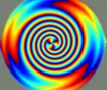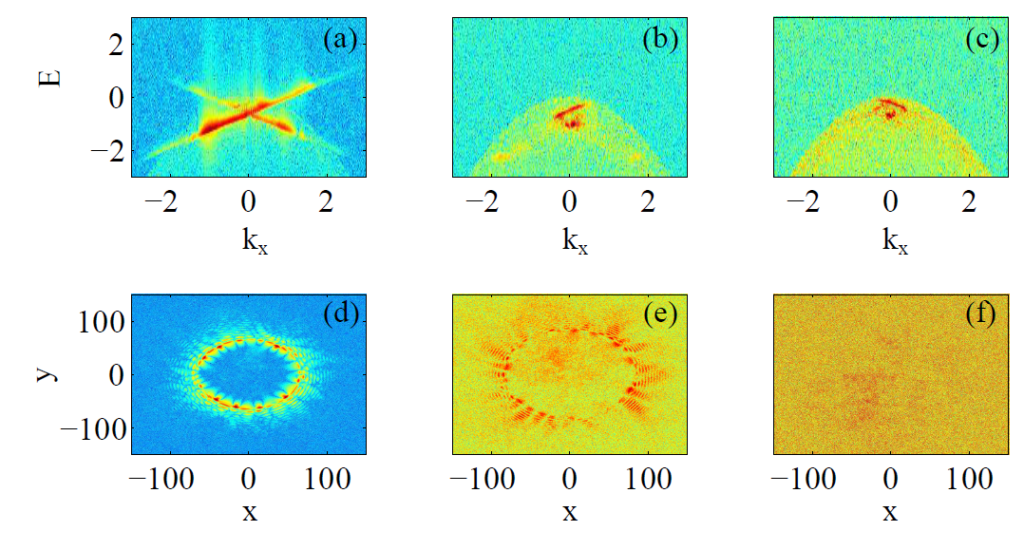# Quantum Simulation of Rainbow GravityRainbow gravity modifies general relativity by introducing an energy dependent metric, which is expected to have a role in the quantum theory of black holes and in quantum gravity at Planck energy scale. We show that rainbow gravity can be simulated in the laboratory by nonlinear waves in nonlocal media, as those occurring in Bose-condensed gases and nonlinear optics. We reveal that at a classical level, a nonlocal nonlinear Schr\”odinger equation may emulate the curved space time in proximity of a rotating black hole as dictated by the rainbow gravity scenario. We also demonstrate that a fully quantized analysis is possible. By the positive $\mathcal{P}$-representation, we study superradiance and show that the instability of a black-hole and the existence of an event horizon are inhibited by an energy dependent metric. Our results open the way to a number of fascinating experimental tests of quantum gravity theories and quantum field theory in curved manifolds, and also demonstrate that these theories may be novel tools for open problems in nonlinear quantum physics.

The picture below shows spectra and configuration of particles trapped in a quantum simulation of a black-hole.Braidotti and Conti, in ArXiv:1708.02623